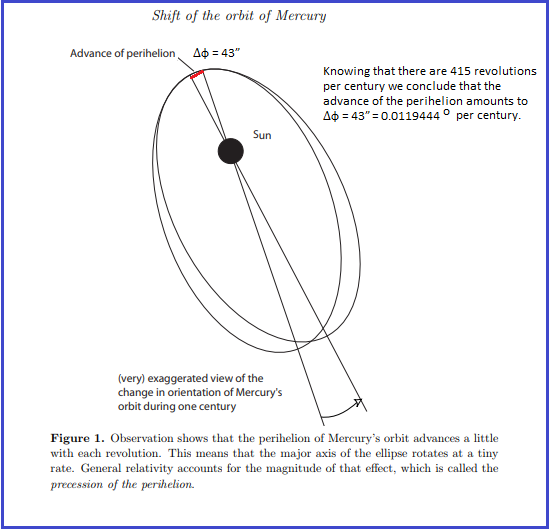Planet Initial Position Xo:

Planet Initial Position Yo:

Planet Initial Velocity Vox:

Planet Initial Velocity Voy:

Number of Iterations:

Time interval delta t:

One Data Set Example :Planet's orbit output is at the bottom of page.

Xo=1, Yo=0, Vox=0, Voy=0.37, No. Iterations=22600, delta t=0.01

This simualation was purely built based on classical Newton's gravitational law. What we can learn from this simulation is: With specific initial position and initial velocity, a planet can have an unusual orbit like Mercury's under purely effect of Newton's Law of Universal Gravitation. This confirmed that the precession of Mercury's perihelion follows Newton's gravitational law!

For one revolution :

Darker color means: The Planet moving slower. Lighter color means: The Planet moving faster

Orbit of the Planet around the Sun :